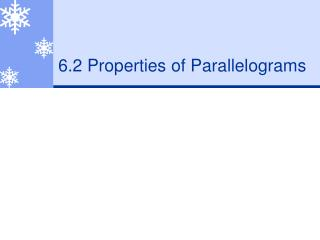DownloadDownload Presentation6.2 Properties of Parallelograms

# 6.2 Properties of Parallelograms

Download Presentation## 6.2 Properties of Parallelograms

- - - - - - - - - - - - - - - - - - - - - - - - - - - E N D - - - - - - - - - - - - - - - - - - - - - - - - - - -
##### Presentation Transcript

1. 6.2 Properties of Parallelograms

2. Theorems • If a quadrilateral is a parallelogram, then its opposite sides are congruent. • If a quadrilateral is a parallelogram, then its opposite angles are congruent. Q R S P

3. Theorems • If a quadrilateral is a parallelogram, then its consecutive angles are supplementary. m<P + m<Q = 180° m<Q + m<R = 180° m<R + m<S = 180° m<S + m<P = 180° Q R S P

4. Using Properties of Parallelograms • PQRS is a parallelogram. Find the angle measure. • m< R • m< Q Q 70 ° R 70 ° + m < Q = 180 ° m< Q = 110 ° 70° P S

5. Using Algebra with Parallelograms • PQRS is a parallelogram. Find the value of h. P Q 3h 120° S R

6. Theorems • If a quadrilateral is a parallelogram, then its diagonals bisect each other. R Q M P S

7. Using properties of parallelograms • FGHJ is a parallelogram. Find the unknown length. • JH • JK 5 5 F G 3 3 K J H

8. Examples • Use the diagram of parallelogram JKLM. Complete the statement. LM K L NK <KJM N <LMJ NL MJ J M

9. Find the measure in parallelogram LMNQ. • LM • LP • LQ • QP • m<LMN • m<NQL • m<MNQ • m<LMQ 18 8 L M 9 110° 10 10 9 P 70° 8 32° 70 ° Q N 18 110 ° 32 °